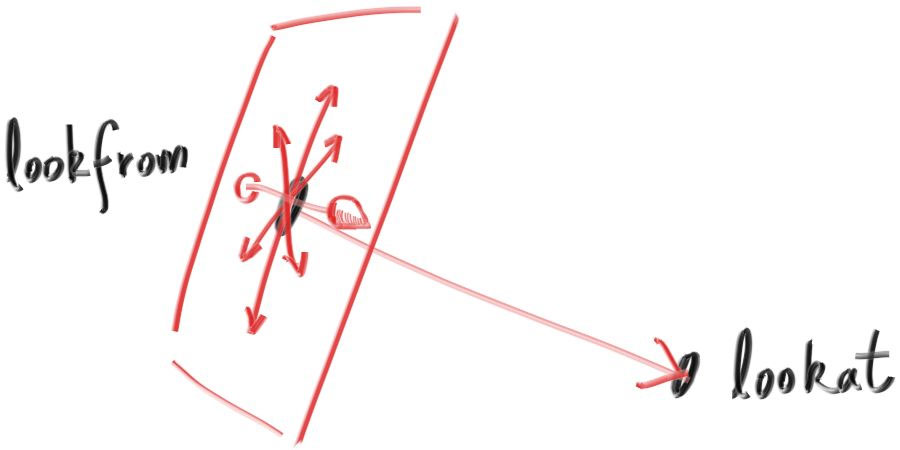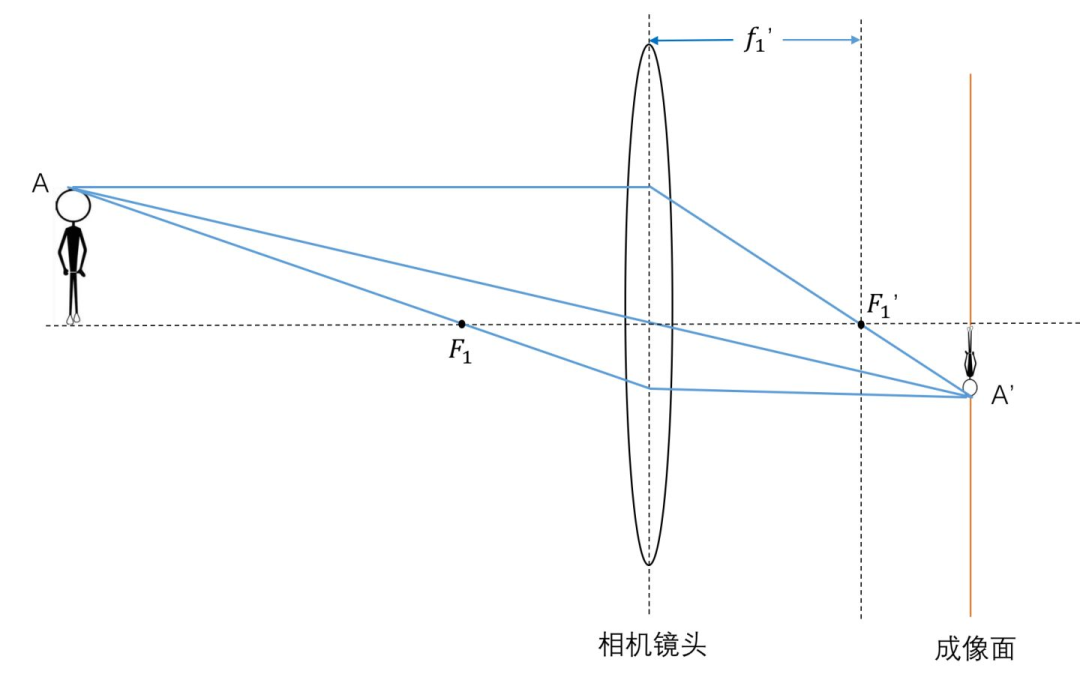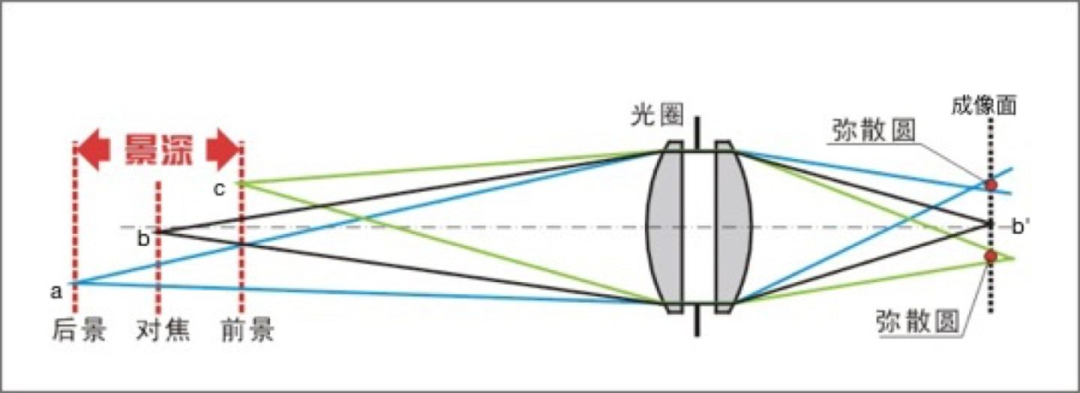# 相机类的实现

## 相机的定位#pragma once
#include "rtow.h"

// 这里的 f8 就是 double （八个字节的 float）
class camera {
public:
camera(vec3 lookfrom, vec3 lookat, vec3 vup = vec3(0, 1, 0), f8 vfov = 90,
f8 asp_ratio = 16.0 / 9.0, f8 aperture = 0, f8 foc_len = 1) {
f8 half_hei = tan(deg_fov / 2);  // 对边比临边，但是临边是 1
f8 half_wid = half_hei * asp_ratio;

cam_z = (lookfrom - lookat).unit_vec();
// z 和镜头指向位置是反的
cam_x = cross(vup, cam_z).unit_vec();  // 同时和 vup，z 垂直
cam_y = vup.unit_vec();

horizon = 2 * half_wid * cam_x * foc_len; // 焦平面的横竖边框
vertic = 2 * half_hei * cam_y * foc_len;

orig = lookfrom;

lower_left_corner = orig - horizon / 2 - vertic / 2 - cam_z * foc_len; // 焦平面的左下角

}

inline ray get_ray(f8 x, f8 y) const {
// x 和 y 的范围：[0, 1]
// 相机传感器的像素点坐标
vec3 rd = len_radius * rand_unit_disk();
vec3 offset = cam_x * rd.x() + cam_y * rd.y();

ray r;
r.orig = orig + offset;
r.dir = lower_left_corner + x * horizon + y * vertic -
orig - offset;
// 产生一个从 orig + offset 到对应像素的向量
// 因为 ray 对应的就是 orig + t * dir
return r;
}

vec3 orig;               // 摄像机的位置
vec3 lower_left_corner;  // 画面的左下角
vec3 horizon, vertic;    // 画面的尺寸（或者说离相机 foc_len 的平面大小）
vec3 cam_x, cam_y, cam_z;// 相机朝向
};f8 deg_fov = deg2rad(vfov);
f8 half_hei = tan(deg_fov / 2);  // 对边比临边，但是临边是 1
f8 half_wid = half_hei * asp_ratio;

cam_z = (lookfrom - lookat).unit_vec();
// z 和镜头指向位置是反的
cam_x = cross(vup, cam_z).unit_vec();  // 同时和 vup，z 垂直
cam_y = vup.unit_vec();
• cam_z 表示一个从 lookatloofrom 的方向，这个方向和相机实际拍摄的位置是相反的。
• cam_x 的计算用到了向量的叉乘，在三维空间中，如果 $u = v \times w$ 那么 $u$ 就是同时垂直于 $v$$w$ 的，当然符合这个条件的向量有两个，可以用右手定则确定，这里就不赘述了。根据前面的这个定义，可以得出 cam_x 同时和 cam_zvup（也就是 cam_z） 垂直。
• cam_y 就是 vup 的单位向量。

https://www.cnblogs.com/qilinzi/archive/2013/05/09/3068158.html

## 相机景深的实现

### 现实中的景深1. 同一点发出的各个方向的光线，经过镜头后必定汇聚于同一点
2. 同一平面上的不同点发出的光线，经过镜头后，汇聚于不同点### 实际实现